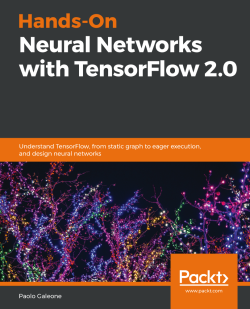### Hands-On Neural Networks with TensorFlow 2.04 (1 reviews total)
By Paolo Galeone
• FREE Subscribe Access now
• \$39.99 Print + eBook
• \$31.99 eBook
• Constantly updated with 100+ new titles each month
• Breadth and depth in over 1,000+ technologies

TensorFlow, the most popular and widely used machine learning framework, has made it possible for almost anyone to develop machine learning solutions with ease. With TensorFlow (TF) 2.0, you'll explore a revamped framework structure, offering a wide variety of new features aimed at improving productivity and ease of use for developers.

This book covers machine learning with a focus on developing neural network-based solutions. You'll start by getting familiar with the concepts and techniques required to build solutions to deep learning problems. As you advance, you’ll learn how to create classifiers, build object detection and semantic segmentation networks, train generative models, and speed up the development process using TF 2.0 tools such as TensorFlow Datasets and TensorFlow Hub.

By the end of this TensorFlow book, you'll be ready to solve any machine learning problem by developing solutions using TF 2.0 and putting them into production.

Publication date:
September 2019
Publisher
Packt
Pages
358
ISBN
9781789615555

# What is Machine Learning?

Machine learning (ML) is an artificial intelligence branch where we define algorithms, with the aim of learning about a model that describes and extracts meaningful information from data.

Exciting applications of ML can be found in fields such as predictive maintenance in industrial environments, image analysis for medical applications, time series forecasting for finance and many other sectors, face detection and identification for security purposes, autonomous driving, text comprehension, speech recognition, recommendation systems, and many other applications of ML are countless, and we probably use them daily without even knowing it!

Just think about the camera application on your smartphone— when you open the app and you point the camera toward a person, you see a square around the person's face. How is this possible? For a computer, an image is just a set of three stacked matrices. How can an algorithm detect that a specific subset of those pixels represents a face?

There's a high chance that the algorithm (also called a model) used by the camera application has been trained to detect that pattern. This task is known as face detection. This face detection task can be solved using a ML algorithm that can be classified into the broad category of supervised learning.

ML tasks are usually classified into three broad categories, all of which we are going to analyze in the following sections:

• Supervised learning
• Unsupervised learning
• Semi-supervised learning

Every group has its peculiarities and set of algorithms, but all of them share the same goal: learning from data. Learning from data is the goal of every ML algorithm and, in particular, learning about an unknown function that maps data to the (expected) response.

The dataset is probably the most critical part of the entire ML pipeline; its quality, structure, and size are key to the success of deep learning algorithms, as we will see in upcoming chapters.

For instance, the aforementioned face detection task can be solved by training a model, making it look at thousands and thousands of labeled examples so that the algorithm learns that a specific input corresponds with what we call a face.

The same algorithm can achieve a different performance if it's trained on a different dataset of faces, and the more high-quality data we have, the better the algorithm's performance will be.

In this chapter, we will cover the following topics:

• The importance of the dataset
• Supervised learning
• Unsupervised learning
• Semi-supervised learning

# The importance of the dataset

Since the concept of the dataset is essential in ML, let's look at it in detail, with a focus on how to create the required splits for building a complete and correct ML pipeline.

dataset is nothing more than a collection of data. Formally, we can describe a dataset as a set of pairs,, whereis the i-th example andis its label, with a finite cardinality,:A dataset has a finite number of elements, and our ML algorithm will loop over this dataset several times, trying to understand the data structure, until it solves the task it is asked to address. As shown in Chapter 2, Neural Networks and Deep Learning, some algorithms will consider all the data at once, while other algorithms will iteratively look at a small subset of the data at each training iteration.

A typical supervised learning task is the classification of the dataset. We train a model on the data, making it learn that a specific set of features extracted from the example(or the example,, itself) corresponds to a label,It is worth familiarizing yourself with the concept of datasets, dataset splits, and epochs from the beginning of your journey into the ML world so that you are already familiar with these concepts when we talk about them in the chapters that follow.

Right now, you already know, at a very high level, what a dataset is. But let's dig into the basic concepts of a dataset split. A dataset contains all the data that's at your disposal. As we mentioned previously, the ML algorithm needs to loop over the dataset several times and look at the data in order to learn how to solve a task (for example, the classification task).

If we use the same dataset to train and test the performance of our algorithm, how can we guarantee that our algorithm performs well, even on unseen data? Well, we can't.

The most common practice is to split the dataset into three parts:

• Training set: The subset to use to train the model.
• Validation set: The subset to measure the model's performance during the training and also to perform hyperparameter tuning/searches.
• Test set: The subset to never touch during the training or validation phases. This is used only to run the final performance evaluation.

All three parts are disjoint subsets of the dataset, as shown in the following Venn diagram: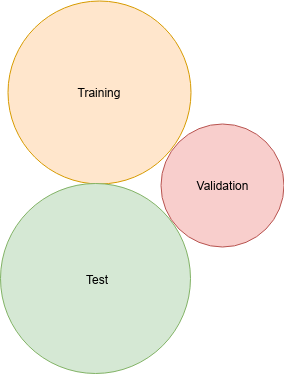Venn diagram representing how a dataset should be divided no overlapping among the training, validation, and test sets is required

The training set is usually the bigger subset since it must be a meaningful representation of the whole dataset. The validation and test sets are smaller and generally the same size—of course, this is just something general; there are no constraints about the dataset's cardinality. In fact, the only thing that matters is that they're big enough for the algorithm to be trained on and represented.

We will make our model learn from the training set, evaluate its performance during the training process using the validation set, and run the final performance evaluation on the test set: this allows us to correctly define and train supervised learning algorithms that could generalize well, and therefore work well even on unseen data.

An epoch is the processing of the entire training set that's done by the learning algorithm. Hence, if our training set has 60,000 examples, once the ML algorithm uses all of them to learn, then an epoch is passed.

One of the most well-known datasets in the ML domain is the MNIST dataset. MNIST is a dataset of labeled pairs, where every example is a 28 x 28 binary image of a handwritten digit, and the label is the digit represented in the image.

However, we are not going to use the MNIST dataset in this book, for several reasons:

• MNIST is too easy. Both traditional and recent ML algorithms can classify every digit of the dataset almost perfectly (> 97% accuracy).
• MNIST is overused. We're not going to make the same applications with the same datasets as everyone else.
• MNIST cannot represent modern computer vision tasks.

The preceding reasons come from the description of a new dataset, called fashion-MNIST, which was released in 2017 by the researchers at Zalando Research. This is one of the datasets we are going to use throughout this book.

Fashion-MNIST is a drop-in replacement for the MNIST dataset, which means that they both have the same structure. For this reason, any source code that uses MNIST can be started using fashion-MNIST by changing the dataset path.

It consists of a training set of 60,000 examples and a test set of 10,000 examples, just like the original MNIST dataset; even the image format (28 x 28) is the same. The main difference is in the subjects: there are no binary images of handwritten digits; this time, there's grayscale images of clothing. Since they are grayscale and not binary, their complexity is higher (binary means only 0 for background and 255 for the foreground, while grayscale is the whole range [0,255]):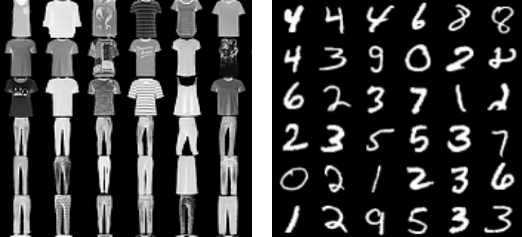Images sampled from the fashion-MNIST dataset on the left and from the MNIST dataset on the right. It's worth noting how the MNIST dataset is simpler since it's a binary images dataset, while the fashion-MNIST dataset is more complex because of the grayscale palette and the inherent complexity of the dataset elements.

A dataset such as fashion-MNIST is a perfect candidate to be used in supervised learning algorithms since they need annotated examples to be trained on.

Before describing the different types of ML algorithms, it is worth becoming familiar with the concept of n-dimensional spaces, which are the daily bread of every ML practitioner.

# n-dimensional spaces-dimensional spaces are a way of modeling datasets whose examples haveattributes each.

Every example,, in the dataset is entirely described by itsattributes,:Intuitively, you can think about an example such as a row in a database table where the attributes are the columns. For example, an image dataset like the fashion-MNIST is a dataset of elements each with 28 x 28 = 284 attributes—there are no specific column names, but every column of this dataset can be thought of as a pixel position in the image.

The concept of dimension arises when we start thinking about examples such as points in an n-dimensional space that are uniquely identified by their attributes.

It is easy to visualize this representation when the number of dimensions is less than or equal to 3, and the attributes are numeric. To understand this concept, let's take a look at the most common dataset in the data mining field: the Iris dataset.

What we are going to do here is explorative data analysis. Explorative data analysis is good practice when you're starting to work with a new dataset: always visualize and try to understand the data before thinking about applying ML to it.

The dataset contains three classes of 50 instances each, where each class refers to a type of Iris plant. The attributes are all continuous, except for the label/class:

• Sepal length in cm
• Sepal width in cm
• Petal length in cm
• Petal width in cm
• Class—Iris Setosa, Iris Versicolor, Iris Virginica

In this small dataset, we have four attributes (plus the class information), which means we have four dimensions that are already difficult to visualize all at once. What we can do to explore the dataset is pick pairs of features (sepal width, sepal length) and (petal width, petal length) and draw them in a 2D plane in order to understand how a feature is related (or not) with another and maybe find out whether there are some natural partitions in the data.

Using an approach such as visualizing the relation between two features only allows us to do some initial consideration on the dataset; it won't help us in a more complex scenario where the number of attributes is way more and not always numerical.

In the plots, we assign a different color to every class, (Setosa, Versicolor, Virginica) = (blue, green, red):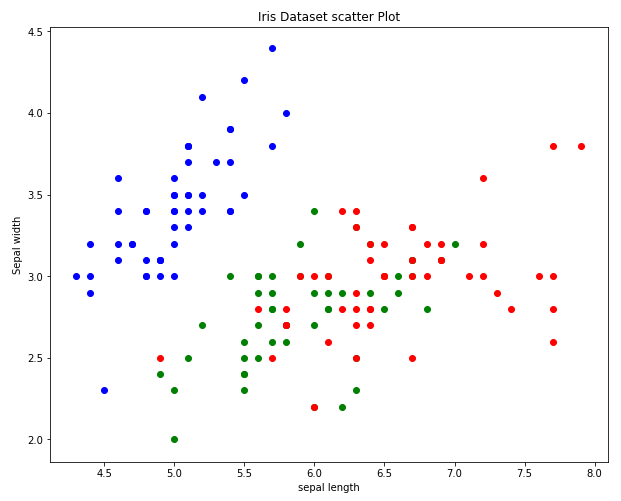Scatter plot of the Iris dataset; every class has a different color and the two dimensions represented are sepal length (x axis) and sepal width (y axis)

As we can see, in this 2D space identified by the attributes (sepal width, sepal length) the blue dots are all close together, while the two other classes are still blended. All we can conclude by looking at this graph is that there could be a positive correlation between the sepal length and width of the Iris setosa, but nothing else. Let's look at the petal relation: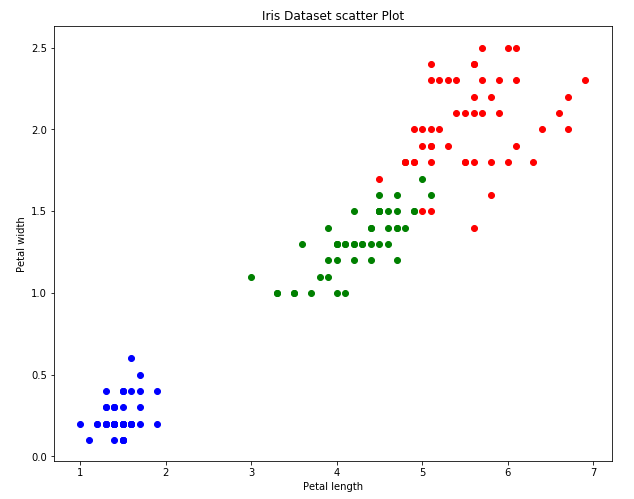Scatter plot of the Iris dataset; every class has a different color and the two dimensions represented are petal length (x axis) and petal width (y axis).

This plot shows us that there are three partitions in this dataset. To find them, we can use the petal width and length attributes.

The goal of classification algorithms is to get them to learn how to identify what features are discriminative in order to learn a function so that they can correctly separate elements of different classes. Neural networks have proven to be the right tool to use to avoid doing feature selection and a lot of data preprocessing: they're so robust to the noise that they almost removed the need for data cleaning.

Warning: This is only valid on massive datasets, where the noise is overwhelmed by the correct data—for small datasets, it is always better to look at the features by plotting them and helping the ML algorithm by giving only the significant features as input.

The Iris dataset is the most straightforward dataset we could have used to describe an n-dimensional space. If we jump back to the fashion-MNIST dataset, things become way more interesting.

A single example has 784 features: how can we visualize a 784-dimensional space? We can't!

The only thing we can do is perform a dimensionality reduction technique in order to reduce the number of dimensions that are needed for visualization and have a better understanding of the underlying data structure.

One of the simplest data reduction techniques—and usually meaningless on high-dimensional datasets—is the visualization of randomly picked dimensions of the data. We did it for the Iris dataset: we just chose two random dimensions among the four available and plotted the data in the 2D plane. Of course, for low-dimensional space, it could be helpful, but for a dataset such as fashion-MNIST, it is a complete waste of time. There are better dimensionality reduction techniques, such as Principal Component Analysis (PCA) or t-distributed Stochastic Neighbor Embedding (t-SNE), that we won't cover in detail in this book, since the data visualization tool we are going to use in the upcoming chapters, that is, TensorBoard, already implements these algorithms for us.

Moreover, there are specific geometrical properties that don't work as we expect them to when we're working in high-dimensional spaces: this fact is called the curse of dimensionality. In the next section, we'll see how a simple geometrical example can be used to show how the Euclidean distances work differently as the number of dimensions increases.

# The curse of dimensionality

Let's take a hypercube unitarywith a center ofin a-dimensional space.

Let's also take a-dimensional hypersphere, withcentered on the origin of the space,. Intuitively, the center of the hypercube,, is inside the sphere. Is this true for every value of?

We can verify this by measuring the Euclidean distance between the hypercube center and the origin:Since the radius of the sphere is 1 in any dimension, we can conclude that, for a value of D greater than 4, the hypercube center is outside the hypersphere.

With the curse of dimensionality, we refer to the various phenomena that arise only when we're working with data in high-dimensional spaces that do not occur in low-dimensional settings such as the 2D or 3D space.

In practice, as the number of dimensions increases, some counterintuitive things start happening; this is the curse of dimensionality.

Now, it should be clearer that working within high-dimensional spaces is not easy and not intuitive at all. One of the greatest strengths of deep neural networks—which is also one of the reasons for their widespread use—is that they make tractable problems in high dimensional spaces, thereby reducing dimensionality layer by layer.

The first class of ML algorithms we are going to describe is the supervised learning family. These kinds of algorithms are the right tools to use when we aim to find a function that's able to separate elements of different classes in an n-dimensional space.

# Supervised learning

Supervised learning algorithms work by extracting knowledge from a knowledge base (KB), that is, the dataset that contains labeled instances of the concept we need to learn about.

Supervised learning algorithms are two-phase algorithms. Given a supervised learning problem—let's say, a classification problem—the algorithm tries to solve it during the first phase, called the training phase, and its performance is measured in the second phase, called the testing phase.

The three dataset splits (train, validation, and test), as defined in the previous section, and the two-phase algorithm should sound an alarm: why do we have a two-phase algorithm and three dataset splits?

Because the first phase (should—in a well-made pipeline) uses two datasets. In fact, we can define the stages:

• Training and validation: The algorithm analyzes the dataset to generate a theory that is valid for the data it has been trained on, but also for items it has never seen.
The algorithm, therefore, tries to discover and generalize a concept that bonds the examples with the same label, with the examples themselves.
Intuitively, if you have a labeled dataset of cats and dogs, you want your algorithm to distinguish between them while being able to be robust to the variations that the examples with the same label can have (cats with different colors, positions, backgrounds, and so on).
At the end of every training epoch, a performance evaluation using a metric on the validation set should be performed to select the model that reached the best performance on the validation set and to tune the algorithm hyperparameters to achieve the best possible result.
• Testing: The learned theory is applied to labeled examples that were never seen during the training and validation phases. This allows us to test how the algorithm performs on data that has never been used to train or select the model hyperparameters—a real-life scenario.

Supervised learning algorithms are a broad category, and all of them share the need for having a labeled dataset. Don't be fooled by the concept of a label: it is not mandatory for the label to be a discrete value (cat, dog, house, horse); in fact, it can also be a continuous value. What matters is the existence of the association (example, value) in the dataset. More formally, the example is a predictor variable, while the value is the dependent variable, outcome, or target variable.

Depending on the type of the desired outcome, supervised learning algorithms can be classified into two different families: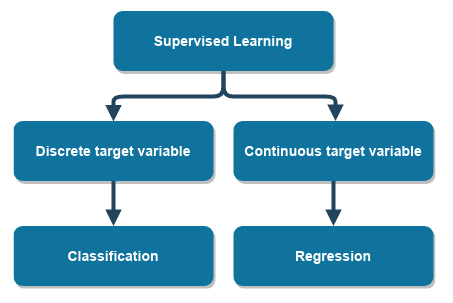The supervised learning family—the target variable defines the problem to solve
• Classification: Where the label is discrete, and the aim is to classify the example and predict the label. The classification algorithm's aim is to learn about classification boundaries. These boundaries are functions that divide the space where the examples live into regions.
• Regression: Where the target variable is continuous, and the aim is to learn to regress a continuous value given an example.
A regression problem that we will see in the upcoming chapters is the regression of the bounding box corner coordinates around a face. The face can be anywhere in the input image, and the algorithm has learned to regress the eight coordinates of the bounding box.

Parametric and non-parametric algorithms are used to solve classification and regression problems; the most common non-parametric algorithm is the k-NN algorithm. This is used to introduce the fundamental concepts of distances and similarities: concepts that are at the basis of every ML application. We will cover the k-NN algorithm in the next section.

# Distances and similarities – the k-NN algorithm

The k-NN algorithm's goal is to find elements similar to a given one, rank them using a similarity score, and return the top-k similar elements (the first k elements, sorted by similarity) found.

To do this, you need to measure the similarity that's required for a function that assigns a numerical score to two points: the higher the score, the more similar the elements should be.

Since we are modeling our dataset as a set of points in an n-dimensional space, we can use anynorm, or any other score function, even if it's not a metric, to measure the distance between two points and consider similar elements that are close together and dissimilar elements that are far away. The choice of the norm/distance function is entirely arbitrary, and it should depend on the topology of the n-dimensional space (that is why we usually reduce the dimensionality of the input data, and we try to measure the distances in lower dimensional space—so the curse of dimensionality gives us less trouble).

Thus, if we want to measure the similarity of elements in a dataset with dimensionality D, given a point, p, we have to measure and collect the distance from p to every other point, q:The preceding example shows the general scenario of computing the generic p norm on the distance vector that connects p and q. In practice, setting p=1 gives us the Manhattan distance, while setting p=2 gives us the Euclidean distance. No matter what distance is chosen, the algorithm works by computing the distance function and sorting by closeness as a measure of similarity.

When k-NN is applied to a classification problem, the point, p, is classified by the vote of its k neighbors, where the vote is their class. Thus, an object that is classified with a particular class depends on the class of the elements that surround it.

When k-NN is applied to regression problems, the output of the algorithm is the average of the values of the  k-NN.

k-NN is only one among the various non-parametric models that has been developed over the years; however, parametric models usually show better performance. We'll look at these in the next section.

# Parametric models

The ML models we are going to describe in this book are all parametric models: this means that a model can be described using a function, where the input and output are known (in the case of supervised learning, it is clear), and the aim is to change the model parameters so that, given a particular input, the model produces the expected output.

Given an input sample,, and the desired outcome,, an ML model is a parametric function,, whereis the set of model parameters to change during the training in order to fit the data (or in other words, generating a hypothesis).

The most intuitive and straightforward example we can give to clarify the concept of model parameters is linear regression.

Linear regression attempts to model the relationship between two variables by fitting a linear equation to observed data.

Linear regression models have the following equation:Here,is the independent variable andis the dependent one. The parameter,, is the scale factor, coefficient, or slope, andis the bias coefficient or intercept.

Hence, the model parameters that must change during the training phase are.

We're talking about a single example in the training set, but the line should be the one that fits all the points of the training set the best. Of course, we are making a strong assumption about the dataset: we are using a model that, due to its nature, models a line. Due to this, before attempting to fit a linear model to the data, we should first determine whether or not there is a linear relationship between the dependent and independent variables (using a scatter plot is usually useful).

The most common method for fitting a regression line is the method of least squares. This method calculates the best-fitting line for the observed data by minimizing the sum of the squares of the vertical deviations from each data point to the line (if a point lies on the fitted line exactly, then its vertical deviation is 0). This relationship between observed and predicted data is what we call the loss function, as we will see in Chapter 2Neural Networks and Deep Learning.

The goal of the supervised learning algorithm is, therefore, to iterate over the data and to adjust theparameters iteratively so thatcorrectly models the observed phenomena.

However, when using more complex models (with a considerable number of adjustable parameters, as in the case of neural networks), adjusting the parameters can lead to undesired results.

If our model is composed of just two parameters and we are trying to model a linear phenomenon, there are no problems. But if we are trying to classify the Iris dataset, we can't use a simple linear model since it is easy to see that the function we have to learn about to separate the different classes is not a simple line.

In cases like that, we can use models with a higher number of trainable parameters that can adjust their variables to almost perfectly fit the dataset. This may sound perfect, but in practice, it is not something that's desirable. In fact, the model is adapting its parameters only to fit the training data, almost memorizing the dataset and thus losing every generalization capability.

This pathological phenomenon is called overfitting, and it happens when we are using a model that's too complex to model a simple event. There's also an opposite scenario, called underfitting, that occurs when our model is too simple for the dataset and therefore is not able to capture all the complexity of the data.

Every ML model aims to learn, and will adapt its parameters so that it's robust to noise and generalize, which means to find a suitable approximate function representing the relationship between the predictors and the response: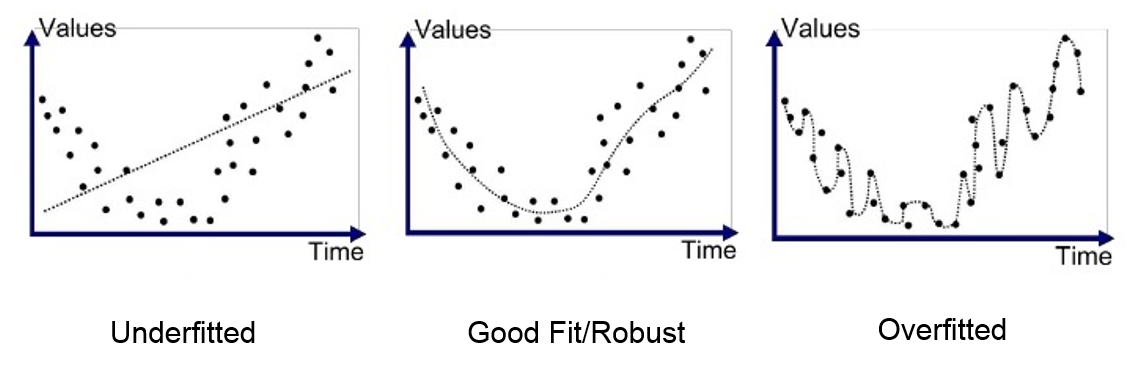The dashed line represents the model's prediction. Underfitting, on the left, is a model with very poor generalization performance and therefore is unable to learn a good dataset approximation. The center image represents a good model that can generalize well in contrast to the model on the right that memorized the training set, overfitting it.

Several supervised learning algorithms have been developed over the years. This book, however, will focus on the ML model that demonstrated to be more versatile and that can be used to solve almost any supervised, unsupervised, and semi-supervised learning task: neural networks.

During the explanation of the training and validation phases, we talked about two concepts we haven't introduced yet—hyperparameters and metrics:

• Hyperparameters: We talk about hyperparameters when our algorithm, which is to be fully defined, requires values to be assigned to a set of parameters. We call the parameters that define the algorithm itself hyperparameters. For example, the number of neurons in a neural network is a hyperparameter.
• Metrics: The functions that give the model prediction. The expected output produces a numerical score that measures the goodness of the model.

Metrics are crucial components in every ML pipeline; they are so useful and powerful that they deserve their own section.

# Measuring model performance – metrics

Evaluating a supervised learning algorithm during the evaluation and testing phases is an essential part of any well-made ML pipeline.

Before we describe the various metrics that are available, there's one last thing that's worth noting: measuring the performance of a model is something that we can always do on every dataset split. During the training phase, usually at the end of every training epoch, we can measure the performance of the algorithm on the training set itself, as well as the validation set. Plotting how the curves change during the training and analyzing the relationships between the validation and training curve allow us to quickly identify the previously described pathological conditions of an ML model—overfitting and underfitting.

Supervised learning algorithms have the significant advantage of having the expected outcome of the algorithm inside the dataset, and all the metrics hereby presented use the label information to evaluate "how well" the model performs.

There are metrics to measure the performance of classifiers and metrics to measure the performance of regressors; it is clear that it wouldn't make any sense to treat a classifier in the same way as a regressor, even if both are members of the supervised learning algorithm family.

The first metric and the most used metric for evaluating a supervised learning algorithm's performance is accuracy.

# Using accuracy

Accuracy is the ratio of the number of correct predictions made to the number of all predictions made.

Accuracy is used to measure classification performance on multiclass classification problems.

Givenas the label andas the prediction, we can define the accuracy of the i-th example as follows:Therefore, for a whole dataset with N elements, the mean accuracy over all the samples is as follows:We have to pay attention to the structure of the dataset, D, when using this metric: in fact, it works well only when there is an equal number of samples belonging to each class (we need to be using a balanced dataset).

In the case of an unbalanced dataset or when the error in predicting that an incorrect class is higher/lower than predicting another class, accuracy is not the best metric to use. To understand why, think about the case of a dataset with two classes only, where 80% of samples are of class 1, and 20% of samples are of class 2.

If the classifier predicts only class 1, the accuracy that's measured in this dataset is 0.8, but of course, this is not a good measure of the performance of the classifier, since it always predicts the same class, no matter what the input is. If the same model is tested on a test set with 40% of samples from class 1 and the remaining ones of class 2, the measurement will drop down to 0.4.

Remembering that metrics can be used during the training phase to measure the model's performance, we can monitor how the training is going by looking at the validation accuracy and the training accuracy to detect if our model is overfitting or underfitting the training data.

If the model can model the relationships present in the data, the training accuracy increases; if it doesn't, the model is too simple and we are underfitting the data. In this case, we have to use a complex model with a higher learning capacity (with a more significant number of trainable parameters).

If our training accuracy increases, we can start looking at the validation accuracy (always at the end of every training epoch): if the validation accuracy stops growing or even starts decreasing, the model is overfitting the training data and we should stop the training (this is called an early stop and is a regularization technique).

# Using the confusion matrix

The confusion matrix is a tabular way of representing a classifier's performance. It can be used to summarize how the classifier behaved on the test set, and it can be used only in the case of multi-class classification problems. Each row of the matrix represents the instances in a predicted class, while each column represents the instances in an actual class. For example, in a binary classification problem, we can have the following:

 Samples: 320 Actual: YES Actual: NO Predicted: YES 98 120 Predicted: NO 150 128

It is worth noting that the confusion matrix is not a metric; in fact, the matrix alone does not measure the model's performance, but is the basis for computing several useful metrics, all of them based on the concepts of true positives, true negatives, false positives, and false negatives.

These terms all refer to a single class; this means you have to consider a multiclass classification problem as a binary classification problem when computing these terms. Given a multiclass classification problem, whose classes are A, B, ..., Z, we have, for example, the following:

• (TP) True positives of A: All A instances that are classified as A
• (TN) True negatives of A: All non-A instances that are not classified as A
• (FP) False positives of A: All non-A instances that are classified as A
• (FN) False negatives of A: All A instances that are not classified as A

This, of course, can be applied to every class in the dataset so that we get these four values for every class.

The most important metrics we can compute that have the TP, TN, FP, and FN values are precision, recall, and the F1 score.

# Precision

Precision is the number of correct positives results, divided by the number of positive results predicted:The metric name itself describes what we measure here: a number in the [0,1] range that indicates how accurate the predictions of the classifier are: the higher, the better. However, as in the case of accuracy, the precision value alone can be misleading. High precision only means that, when we predict the positive class, we are precise in detecting it. But this does not mean that we are also accurate when we're not detecting this class.

The other metric that should always be measured to understand the complete behavior of a classifier is known as recall.

# Recall

The recall is the number of correct positive results, divided by the number of all relevant samples (for example, all the samples that should be classified as positive):Just like precision, recall is a number in the [0,1] range that indicates the percentage of correctly classified samples over all the samples of that class. The recall is an important metric, especially in problems such as object detection in images.

Measuring the precision and recall of a binary classifier allows you to tune the classifier's performance, making it behave as needed.

Sometimes, precision is more important than recall, and vice versa. For this reason, it is worth dedicating a short section to the classifier regime.

# Classifier regime

Sometimes, it can be worth putting a classifier in the high-recall regime. This means that we prefer to have more false positives while also being sure that the true positives are detected.

The high-recall regime is often required in computer vision industrial applications, where the production line needs to build a product. Then, at the end of the assembly process, a human controls whether the quality of the complete product reaches the required standard.

The computer vision applications that control the assembly robots usually work in a high-recall regime since the production line needs to have high throughput. Setting the computer vision applications in a high-precision regime would have stopped the line too often, reducing the overall throughput and making the company lose money.

The ability to change the working regime of a classifier is of extreme importance in real-life scenarios, where the classifiers are used as production tools that should adapt themselves to the business decisions.

There are other cases where a high-precision regime is required. In industrial scenarios, there are also processes commanded by computer vision applications that are critical and for this reason, require high accuracy.

In an engine production line, classifiers could be used to decide on which part the camera sees is the correct one to pick and to assemble in the engine. In critical cases like this one, a high-precision regime is required and a high-recall regime is discouraged.

A metric that combines both precision and recall is the F1 score.

# F1 score

The F1 score is the harmonic mean between precision and recall. This number, which is in the [0,1] range, indicates how precise the classifier is (precision) and how robust it is (recall).

The greater the F1 score, the better the overall performance of the model:# Using the area under the ROC curve

The area under the  Receiving Operating Characteristic (ROC) curve is one of the most used metrics for the evaluation of binary classification problems.

Most classifiers produce a score in the [0,1] range and not directly as a classification label. The score must be thresholded to decide the classification. A natural threshold is to classify it as positive when the score is higher than 0.5 and negative otherwise, but this is not always what our application wants (think about the identification of people with a disease).

Varying the threshold will change the performance of the classifier, varying the number of TPs, FPs, TNs, and FNs, and thereby the overall classification performance.

The results of the threshold variations can be taken into account by plotting the ROC curve. The ROC curve takes into account the false positive rate (specificity) and the true positive rate (sensitivity): binary classification problems are a trade-off between these two values. We can describe these values as follows:

• Sensitivity: The true positive rate is defined as the proportion of positive data points that are correctly considered positive, with respect to all the positive data points:• Specificity: The false positive rate is defined as the proportion of negative data points that are considered positive, with respect to all the negative data points:The AUC is the area under the ROC curve, and is obtained by varying the classification threshold: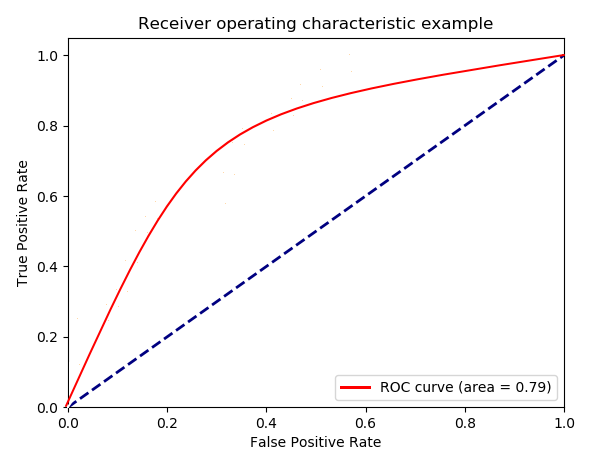ROC curve obtained by varying the classification threshold. The dashed lines represent the expectation for random guessing.

It is clear that both TPR and FPR have values in the [0,1] range, and the graph is drawn by varying the classification threshold of the classifier in order to get different pairs of TPR and FPR for every threshold value. The AUC is in the [0,1] range too and the greater the value, the better the model is.

If we are interested in measuring the performance of a regressor's precision and recall and all the data that was gathered from the confusion matrix is useless, then we have to use other metrics to measure the regression error.

# Mean absolute error

Mean absolute error (MAE) is the average of the absolute difference between the original and the predicted values. Since we are now interested in the measurement of the performance of a regressor, we have to take into account that theandvalues are numerical values:The MAE value has no upper bound, and its lower bound is 0. It should be evident that we want the MAE value to be as close as possible to 0.

MAE gives us an indication of how far the predictions are from the actual output; this metric is easily interpretable since its value is also on the same scale as the original response value.

# Mean squared error

Mean squared error (MSE) is the average of the squared difference between the original and the predicted values:Just like MAE, MSE has no upper bound and its lower bound is 0.

On the contrary, the presence of the square terms makes the metric less easy to interpret.

A good practice to follow is to consider both metrics so that you get as much information as possible about the distribution of the errors.

Therelation holds, and so the following is true:

• If MSE is close to MAE, the regressor makes small errors
• If MSE is close to MAE², the regressor makes large errors

Metrics are probably the most important part of the ML model selection and performance measurement tools: they express relations between the desired output and model output. This relation is fundamental since it is what we want to optimize our model for, as we will see in Chapter 2Neural Networks and Deep Learningwhere we will introduce the concept of the loss function.

Moreover, since the models we are treating in this book are all parametric models, we can measure the metrics during/at the end of the training process and save the model parameters (and by definition, the model) that reached the best validation/test performance.

Using parametric models allows us this kind of flexibility— we can freeze the status of a model when it reaches the desired performance and go ahead with training, changing hyperparameters, and experimenting with different configurations/training strategies, while also having the certainty of having stored a model that already has good performance.

Having metrics and the ability to measure them during the training process, together with the usage of parametric models that can be saved, gives us the power to evaluate different models and save only the one that fits our needs best. This process is called model selection and is fundamental in every well-made ML pipeline.

We've focused on the supervised learning family algorithm a lot, but of course, ML is much more than this (even tough supervised learning algorithms have the best performance when it comes to solving real-life problems).

The next family of algorithms we are briefly going to describe are from the unsupervised learning family.

# Unsupervised learning

In comparison to supervised learning, unsupervised learning does not need a dataset of labeled examples during the training phaselabels are only needed during the testing phase when we want to evaluate the performance of the model.

The purpose of unsupervised learning is to discover natural partitions in the training set. What does this mean? Think about the MNIST dataset—it has 10 classes, and we know this because every example has a different label in the [1,10] range. An unsupervised learning algorithm has to discover that there are 10 different objects inside the dataset and does this by looking at the examples without prior knowledge of the label.

It is clear that unsupervised learning algorithms are challenging compared to supervised learning ones since they cannot rely on the label's information, but they have to discover features and learn about the concept of labels by themselves. Although challenging, their potential is huge since they discover patterns in data that humans can struggle to detect. Unsupervised learning algorithms are often used by decision-makers that need to extract meaning from data.

Just think about the problem of fraud detection: you have a set of transactions, a huge volume of money exchanged between people, and you don't know if there are fraudulent transactions inside them because there are no labels in the real world!

In this scenario, the application of unsupervised learning algorithms could help you find the big natural partition of normal transactions and help you discover the outliers.

Outliers are the points outside, and usually far away, from any partition (also called a cluster) found in the data, or a partition itself with some particular characteristic that makes it different from the normal ones.

Unsupervised learning is, for this reason, used frequently in anomaly detection tasks, and in many different domains: not only fraud detection, but also quality control in images, video streams, streams of datasets coming from sensors in production environments, and much more.

Unsupervised learning algorithms are two-phase algorithms as well:

• Training and validation: Since there are no labels inside the training set (and they should be discarded if present), the algorithm is trained to discover the existing patterns in the data. If there's a validation set, that should contain labels; the model's performance can be measured at the end of every training epoch.
• Testing: A labeled dataset is given in the input to the algorithm (if such a dataset exists) and its results are compared with the label's information. In this phase, we measure the performance of the algorithm using the label's information in order to verify that the algorithm learned to extract patterns from data that humans have also been able to detect.

Working on these examples only, unsupervised learning algorithms are not classified on the basis of the label type (as the supervised learning algorithms), but on what they aim to discover.

Unsupervised learning algorithms can be classified as follows:

• Clustering: The aim is to discover clusters, that is, natural partitions of the data.
• Association: In this case, the aim is to discover rules that describe data and associations between them. These are usually used to give recommendations: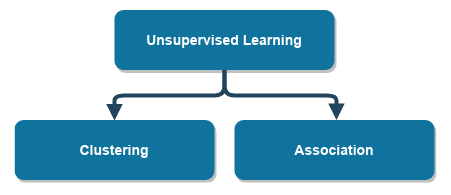The unsupervised learning family—there are two main families of algorithms

The association learning algorithms are powerful tools of the data mining world: they're used to discover rules, such as "if a person is buying butter and bread, they will probably also buy milk". Learning about these rules can be a huge competitive advantage in business. By recalling the previous example, we can say that a store can place butter, bread, and milk together on the same shelf to maximize selling!

During the training phase of a clustering algorithm, we are interested in measuring the performance of the model, just like we do in the supervised learning case. Metrics, in the case of unsupervised learning algorithms, are more complex and task-dependent. What we usually do is exploit additional labels present in the dataset, but that aren't used during the training, and thus reconduct the problem to a supervised learning problem and use the usual metrics.

As in the case of supervised learning, there are parametric and non-parametric models.

Most non-parametric algorithms work by measuring the distance between a data point and every other data point in the dataset; then, they use the distance information to cluster the data space in different regions.

Like in the supervised learning case, a lot of algorithms have been developed over the years to find natural partitions and/or rules in non-labeled datasets. However, neural networks have been applied to solve unsupervised learning tasks and have achieved superior performance and shown to be very flexible. This is another reason why this book only focuses on neural networks.

Unsupervised learning algorithms explicitly require to do not have any label information during the training phase. However, since labels could be present in the datasets, why not take advantage of their presence while still using a ML algorithm to discover other patterns in the data?

# Semi-supervised learning

Semi-supervised learning algorithms fall between supervised and unsupervised learning algorithms.

They rely upon the assumption that we can exploit the information of the labeled data to improve the result of unsupervised learning algorithms and vice versa.

Being able to use semi-supervised learning algorithms depends on the available data: if we have only labeled data, we can use supervised learning; if we don't have any labeled data, we must go with unsupervised learning methods. However, let's say we have the following:

• Labeled and unlabeled examples
• Examples that are all labeled with the same class

If we have these, then we can use a semi-supervised approach to solve the problem.

The scenario in which we have all the examples labeled with the same class could look like a supervised learning problem, but it isn't.

If the aim of the classification is to find a boundary that divides at least two regions, how can we define a boundary among regions if we only have a single region?

We can't!

An unsupervised or semi-supervised learning approach is the way to go for these kinds of problems: the algorithm will learn how the input space is partitioned (hopefully, in one single cluster), its shape, and how the data is distributed in the space.

An unsupervised learning approach could be used to learn that there is a single cluster in the data. By using the labels, and thereby switching to a semi-supervised learning approach, we can enforce some additional constraints on the space so that we lean toward a better representation of the data.

Once the unsupervised/semi-supervised learning algorithm has learned about a representation of the data, we can test whether a new example—one that we have never seen during the training process—falls inside the cluster or not. Alternatively, we can calculate a numerical score that tells us "how much" the new example fits inside the learned representation.

Just like the unsupervised learning algorithms, the semi-supervised algorithm has two phases.

# Summary

In this chapter, we went through the ML algorithm families from a general and theoretical point of view. It is essential to have good knowledge of what machine learning is, how algorithms are categorized, what kind of algorithms are used given a certain task, and how to become familiar with all the concepts and the terminology that's used among machine learning practitioners.

In the next chapter, Chapter 2Neural Networks and Deep Learning, we will focus on neural networks. We will understand the strengths of machine learning models, how is it possible to make a network learn, and how, in practice, a model parameter update is performed.

# Exercises

Answering the following questions is of extreme importance: you are building your ML foundations—do not skip this step!

1. Given a dataset of 1,000 labeled examples, what do you have to do if you want to measure the performance of a supervised learning algorithm during the training, validation, and test phases, while using accuracy as the unique metric?
2. What is the difference between supervised and unsupervised learning?
3. What is the difference between precision and recall?
4. A model in a high-recall regime produces more or less false positives than a model in a low recall regime?
5. Can the confusion matrix only be used in a binary classification problem? If not, how can we use it in a multiclass classification problem?
6. Is one-class classification a supervised learning problem? If yes, why? If no, why?
7. If a binary classifier has an AUC of 0.5, what can you conclude from this?
8. Write the formula of precision, recall, F1-score, and accuracy. Why is F1 important? Is there a relationship between accuracy, precision, and recall?
9. The true positive rate and false positive rate are used to plot the ROC curve. What is the ROC curve's purpose, and is there a relationship among the true positive rate/false positive rate and precision/recall? Hint: write the math.
10. What is the curse of dimensionality?
11. What are overfitting and underfitting?
1. What is the learning capacity of a model? Is it related to the condition of overfitting/underfitting?
2. Write the Lp norm formula—is this the only way to measure the distance among points?
3. How can we say that a data point is similar to another data point?
4. What is model selection? Why is it important?